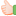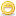# Thread: How do you get 1000 from eight 8's

1. ##How do you get 1000 from eight 8's

Try this:

How will you get 1000 from eight 8's using any of these arithmetic operators

{+,-,*,/,(,)}Reply With Quote

2. ## Re: How do you get 1000 from eight 8's

888+88+8+8+8=1000Reply With Quote

3. ## Re: How do you get 1000 from eight 8's

Good try but not correct... I said each 8 should have atleast 1 operator between them.

here is the answer:

(((8*8)+(8*8))*8)-(8+8+8) = 1000

EnjoyyReply With Quote

4. ## Re: How do you get 1000 from eight 8'sOriginally Posted by PSharma1811I said each 8 should have atleast 1 operator between them.
Hi,

This rule you are telling it now only.

GanesanReply With Quote

5. ## Re: How do you get 1000 from eight 8's

Hey good question.Reply With Quote

6. ## Re: How do you get 1000 from eight 8's

hey man i got a good logic to remember this answer given by P sharma.
here it is,
1000=1024-24
=2^10-24
=((2^6+2^6)*2^3)-(8+8+8)
=((8*8+8*8)*8)-(8+8+8)Reply With Quote

7. ## Re: How do you get 1000 from eight 8's

((8*8)+(8*8)*8-(8+8+8))=1000Reply With Quote

8. ##Re: How do you get 1000 from eight 8's

8888-888/8=1000Reply With Quote

9. ##Re: How do you get 1000 from eight 8's

how do you do it is for my class and i need to find it out.
can you help me?Reply With Quote

10. ## Re: How do you get 1000 from eight 8's

[(8*8)+(8*8)]*8-(8+8+8)Reply With Quote

11. ## Re: How do you get 1000 from eight 8's

(((8*8)+8)+((8+8)/8)+8/8)+((8*8)-(8+8))+(8+8/8))*8=1000Reply With Quote

12. ## Re: How do you get 1000 from eight 8's

(8*8*8)+(8*8*8)-(8+8+8)Reply With Quote

13. ## Re: How do you get 1000 from eight 8's

8888/8.888Reply With Quote

####Posting Permissions

• You may not post new threads
• You may not post replies
• You may not post attachments
• You may not edit your posts
•